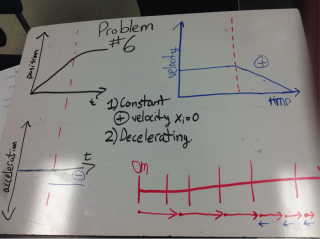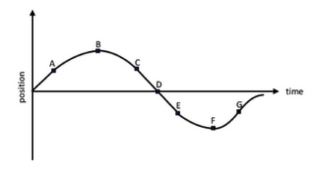# More Representations of Motion for Uniform Acceleration

3 teachers like this lesson
Print Lesson

## Objective

Students will be able to create 5 representations of motion for each situation including written descriptions, motion maps and motion graphs.

#### Big Idea

Students present and explain multiple representations of uniform accelerated motion through multiple group activities.

## Whiteboard Prep Time

25 minutes

The goal of this lesson is for students to interact more with multiple representations of uniform accelerated motion. Students have worked and practiced with the new acceleration graphs and motion maps, but in this lesson my aim is for them to explain the graphs and what is happening to an accelerating object.

To start out class, I ask students to take out their homework from the night before (Worksheet #1 Stacks of Motion Graphs). I ask students to take about 15 minutes to talk with their groups and compare answers. I put a timer on the front projector screen and walk around to make sure that students are focused and so I can answer any questions that they have. My goal is for students to try to talk more to each other before they ask me questions. I will answer questions but I find myself asking "Did you ask everyone in your group yet?" a lot.

After the 15 minutes is up, I tell students that each group will be in charge of whiteboarding one problem and presenting in front of class. I have a whiteboard prepared for the problem that I did as an example in the last class to show them what I expect. I present the problem making sure that I start with the position vs. time graph and written description and then move to the velocity vs. time graph, then acceleration vs. time graph, then the motion map. After I have presented my problem, I ask students if there are any questions. I assign problems by rolling a die and allowing the table group with the rolled number to choose which problem from the packet to whiteboard. I do this so that students have a choice in determining which of the problems that they present.

## Whiteboard Presentations

30 minutes

After students have completed their whiteboards for Worksheet #1 Stacks of Motion Graphs, I have students make sure that each person in the group will be speaking during the presentations by selecting beforehand what each group member will say. Since there are four group members, I suggest that one person explain how they got the written description, motion map and each graph (velocity vs. time and acceleration vs. time). Then, I have the group presenting problem #1 stand up at the front of class and begin the presentations. Student whiteboards look similar to the ones below.In whiteboard presentations, my expectations for students when they are presenting are: provide the answer they found and answer any questions from students and me. My expectations for students when they are not presenting are to check their work with the answer on the whiteboard to see if they agree. If audience members don't agree, I expect them to ask a question to the presenters at the end of the presentation. I want students to ask questions during the presentations and advocate for themselves when they don't understand. I make a goal to ask at least one question for each presentation. If there are problems with what the group is presenting on their whiteboard, I ask questions about that. If there are no problems, I ask questions that I have prepared before hand on WS #1 KEY with Questions.

During the whiteboard sessions, I make sure to emphasize that on the velocity vs. time graphs that a horizontal line or no slope means that the object is at constant velocity and velocity vs. time graphs that have a slope are either accelerating or decelerating. I also make sure to emphasize that acceleration vs. time graphs are always horizontal lines because we are only dealing with situations of constant acceleration.

## End of Discussion Checkpoint

5 minutes

After students have seen the answers to the worksheet, I want to see what they have learned from doing the worksheet and the presentations so I give them End of Discussion Checkpoint #1 for them to complete individually. Since they have had exposure to the multiple representations of motion, I give them two velocity vs. time graphs. I give them one that is constant velocity motion and one that is uniform accelerated motion. Since we just had an entire unit on constant velocity motion I anticipate them getting the first stack correct and struggling more with the second, if they struggle with one of them. After students turn them in, I grade them after this class period and hand them back to students during the next class.

## Worksheet #2: Interpreting Graphs of Accelerated Motion

15 minutes

After students complete their checkpoint, I ask them to work on Worksheet #2: Interpreting Graphs of Accelerated Motion. This worksheet looks at representations of uniform accelerated motion in a different way, by only looking at one graph to determine motion. The first problem has them looking at what is happening to the slope at each point labeled along the curve, as shown below. When students have questions about this problem, I tell them that the best way to look at it is if they draw their slope lines in and see if the slope is getting steeper or less steep. I chose this problem because I want them to see all of the different types of acceleration on a position vs. time graph together so they can begin to see the difference between the curves.The second problem gives a quantitative velocity vs. time graph that guides them to identify the different parts of the mathematical model like slope (acceleration) and y-intercept (initial velocity). I want students to start to see a connection between our previous units on creating mathematical models to the graphs that we are seeing in the current unit. I give them until the end of the period to work on this worksheet and tell them that we will go over it next class with the WS #2 KEY.